# Test Prep Plan - Take a practice test

Take this practice test to check your existing knowledge of the course material. We'll review your answers and create a Test Prep Plan for you based on your results.
How Test Prep Plans work
1
2Based on your results, we'll create a customized Test Prep Plan just for you!
3Study smarter
Study more effectively: skip concepts you already know and focus on what you still need to learn.

# Limits of Functions Chapter Exam

Exam Instructions:

Choose your answers to the questions and click 'Next' to see the next set of questions. You can skip questions if you would like and come back to them later with the yellow "Go To First Skipped Question" button. When you have completed the practice exam, a green submit button will appear. Click it to see your results. Good luck!

### Page 1

#### Question 1 1. Evaluate the limit below.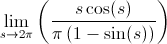#### Question 2 2. Using the squeeze theorem, what is z?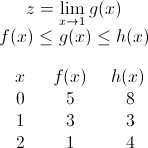#### Question 3 3. Use the given graph and formula to solve for z.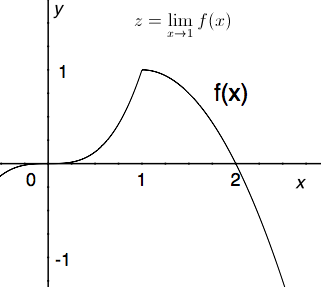#### Question 4 4. Calculate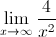#### Question 5 5. Which of the following is true?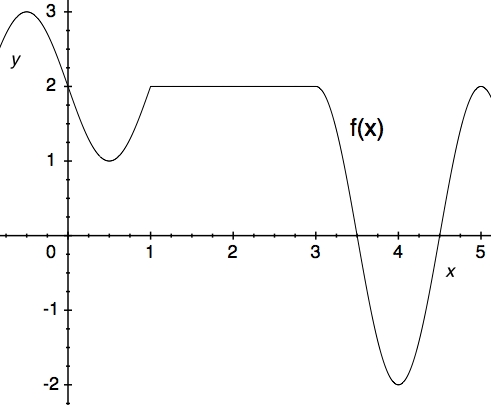### Page 2

#### Question 6 6. Use the given formula to solve for z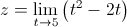#### Question 7 7. Using the given data, predict the likely value of the continuous function z.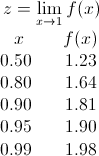#### Question 9 9. Calculate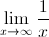#### Question 10 10. Calculate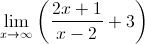### Page 3

#### Question 11 11. Use the graph to solve for z.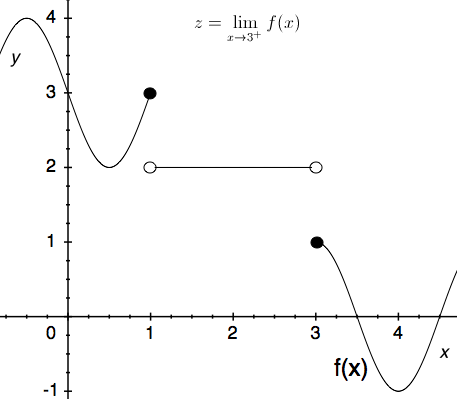#### Question 12 12. Using the given data, solve for the continuous function y.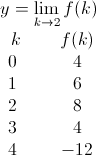#### Question 13 13. The expression below can also be written as: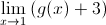### Page 4

#### Question 16 16. Calculate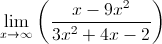#### Question 17 17. Use the graph to solve for z.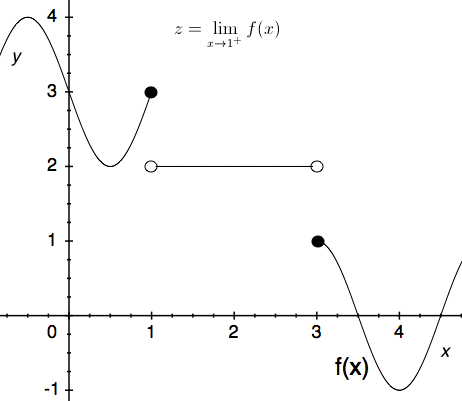#### Question 18 18. Use the given graph and formula to solve for z.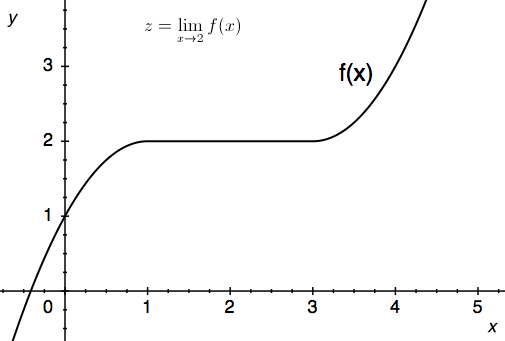#### Question 20 20. Using the squeeze theorem, what is z?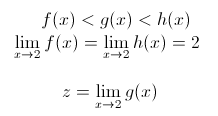### Page 5

#### Question 21 21. The floor function is graphically represented as a stepwise function. What is the limit of f(x) = floor(x) as x approaches 4?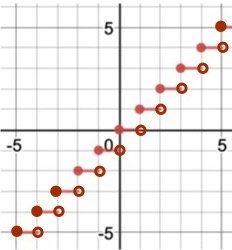#### Question 22 22. The expression below can also be written as: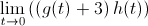#### Question 23 23. Using the squeeze theorem, what is z?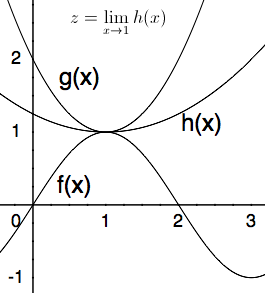#### Question 24 24. Using the squeeze theorem, what is z?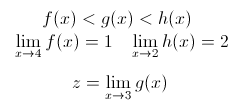#### Question 25 25. The ceiling function is graphically translated as a stepwise function. What is the limit of f(x) = ceil(x) as x approaches 4.5?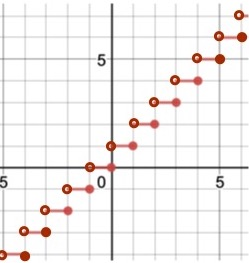### Page 6

#### Question 27 27. Using the graph, find the value of z, then add 1.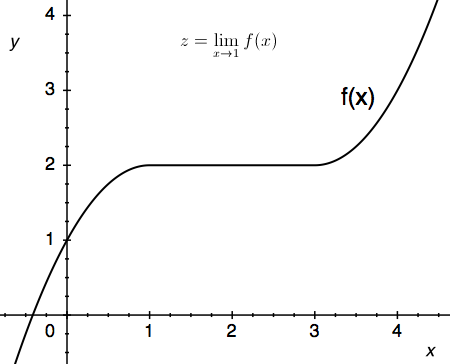#### Question 28 28. Use the graph to solve for z.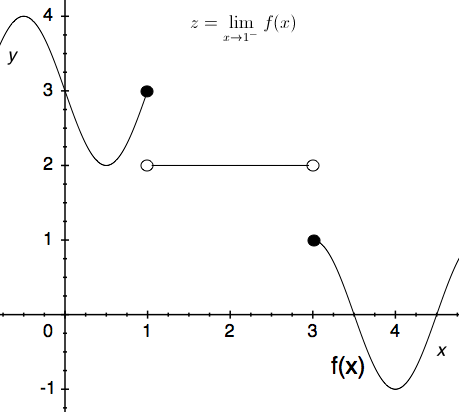#### Question 30 30. Using the squeeze theorem, what is z?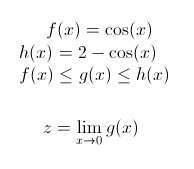#### Limits of Functions Chapter Exam Instructions

Choose your answers to the questions and click 'Next' to see the next set of questions. You can skip questions if you would like and come back to them later with the yellow "Go To First Skipped Question" button. When you have completed the practice exam, a green submit button will appear. Click it to see your results. Good luck!

Support